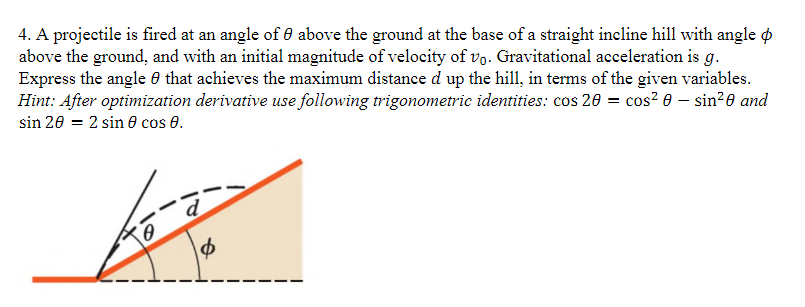Home / Answered Questions / Other / 4-a-projectile-is-fired-at-an-angle-of-above-the-ground-at-the-base-of-a-straight-incline-hill-with--aw325

# (Solved): 4. A Projectile Is Fired At An Angle Of Above The Ground At The Base Of A Straight Incline Hill With...4. A projectile is fired at an angle of above the ground at the base of a straight incline hill with angle â€¢ above the ground, and with an initial magnitude of velocity of vo. Gravitational acceleration is g. Express the angle that achieves the maximum distance d up the hill, in terms of the given variables. Hint: After optimization derivative use following trigonometric identities: cos 20 = cos - sine and sin 20 = 2 sin cos 0. toi

We have an Answer from Expert